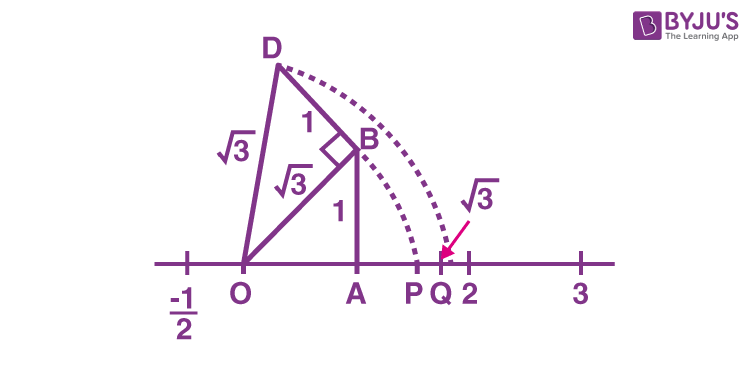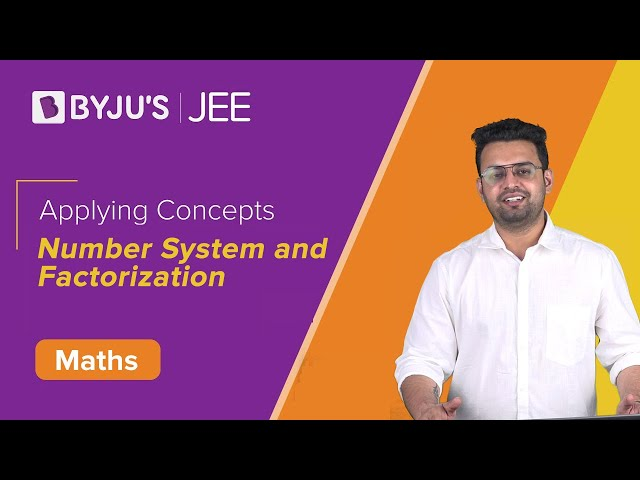# Number System Questions

Number systems questions are provided here with solutions. In Class 9, we will come across the Number System chapter where we learn the basics of different types of numbers and their applications. In number theory, you may have learned about the different classifications of numbers, such as whole and natural numbers, even and odd numbers, etc. Here, we will solve the problems based on rational and irrational numbers. Students can practice the questions and it would be helpful for the students to understand this chapter. Here, we have provided a variety of number system questions and some important questions for practice. Let us learn in brief about each concept covered in this chapter before we solve a question based on it.

Also, read: Number System For Class 9

## Number System Questions with Solutions

 Rational numbers: The numbers represented in the form of p/q, where p and q are integers and q is not equal to 0. Note: There are infinitely many rational numbers between any two given rational numbers.

1. What are the five rational numbers between 1 and 2?

Solution: We need to find 5 rational numbers between 1 and 2

Divide and multiply both the numbers by (5+1)

Hence,

6/6 and 12/6 are rational numbers now.

Therefore, the required rational numbers between 1 and 2 are:

6/6, 7/6, 8/6, 9/6, 10/6, 11/6, 12/6.

 Irrational numbers: A number that cannot be represented in the form p/q.

2. Can we locate √3 on the number line?

Solution: Yes, we can locate it.

Follow the steps to locate it: Construct BD of unit length perpendicular to OB

Then using the Pythagoras theorem, we see that OD = √((√2)2+12) = √3

With centre O and radius OD, using a compass, draw an arc that cuts the number line at the point Q.Terminating and Non-terminating Decimal Expansion For a rational number, the decimal expansion is either terminating or non-terminating recurring. For an irrational number, the decimal expansion is non-terminating and non-recurring.

4. Show that 0.3333…, can be expressed in the form of rational number, i.e. p/q.

Solution: Let x = 0.33333

10 x = 10 × (0.333…) = 3.333…

We can write,

3.3333… = 3 + 0.3333… = 3 + x

Thus,

10 x = 3 + x

9x = 3

x=⅓

5. Write the following in decimal form and mention what expansion it is.

(i) 36/100

(ii) 1/11

Solution:

(i) 36/100 = 0.36

It is terminating.

(ii) 1/11 = 0.09090909…

It is non-terminating and repeating

6. Add 2√2 + 5√3 and √2 – 3√3.

Solution: (2√2 + 5√3) + (√2 – 3√3)

= (2+1)√2+(5-3)√3

= 3√2+2√3

7. Multiply 6√2 by 2√2.

Solution: 6√2 x 2√2

6 x 2 x √2 x √2

= 12 x 2

= 24

8. Rationalise the denominator of 1/√3

Solution: To rationalise the denominator of 1/√3, we need to multiply the numerator and denominator by √3

1/√3 x (√3/√3) = √3/3

9. Rationalise the denominator of √2/(√3-√5).

Solution: Multiply both numerator and denominator by √3+√5

[√2/(√3-√5)] x [(√3+√5)/(√3+√5)]

Numerator = √2(√3+√5)

Denominator = (√3-√5)(√3+√5) = (√3)2-(√5)2 = 3-5 = -2

Therefore,

[√2(√3+√5)]/-2

= [-√2(√3+√5)]/2

 Laws of exponents for real numbers: (i) am.an = am+n (ii) (am)n = amn (iii) am/an = am-n, m>n (iv) ambm = (ab)m

10. Simplify:

(i) 21/3.22/3

(ii) (31/5)4

(iii) 71/3/71/5

(iv) 131/7.171/7

Solution:

(i) 21/3.22/3

= 21/3+⅔

= 23/3

= 2

(ii) (31/5)4

= 34/5

(iii) (71/3)/(71/5)

= 7(1/3)-(1/5)

= 72/15

(iv) 131/7.171/7

= (13.17)1/7

= 2211/7

## Video Lesson

### Number System and Factorisation### Number System Questions for Practice

1. If (p×q) = 6p-4q+3pq, then find the value of [(6×3)+(4×3)]
2. Find out which of the following numbers are prime numbers, given that “p” is a prime number.
• (a)2p  (b)p2 (c)3p (d) p-2 (e) p-3
1. Write down the five rational numbers between 6/5 and 7/5
2. Express the decimal number 1.2343 in the form of a rational number (i.e p/q form)
3. Simplify the expression (22-3). (4+22)

## Frequently Asked Question on the Number System Questions

### What is meant by number system?

In mathematics, a number system is defined as the way of expressing the numbers. The number system provides a distinct way of expressing different types of numbers and it also provides the algebraic structure of the mathematical problem.

### What are the different types of numbers?

The different types of numbers are:
Natural Numbers
Whole numbers
Real Numbers
Rational Numbers
Irrational numbers
Complex numbers

### Why do we use numbers?

The numbers are used to count the surrounding thing. Numbers are used for expressing money, time, date, and so on. Without numbers, we could not be able to understand the value of many things

### What are the four different types of number system?

The four major types of number system are:
Binary number system (base 2 number system)
Octal number system (base 8 number system)
Decimal number system (base 10 number system)
Hexadecimal number system (base 16 number system)

### What are the applications of the number system?

The most common application of the number system is found in computer technology. It uses the binary number system. The base 2 number system is used in the process of digital encoding

#### 1 Comment

1. Harshit

Very nice questions with explaintion